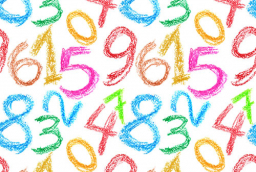# The sum 9

The sum of two integers is 4. Their difference is 8. What are this two integers?Did you find an error or inaccuracy? Feel free to write us. Thank you!Tips to related online calculators
Do you have a system of equations and looking for calculator system of linear equations?